Courses

# Test: Gaseous State- 3

## 30 Questions MCQ Test Physical Chemistry | Test: Gaseous State- 3

Description
This mock test of Test: Gaseous State- 3 for Chemistry helps you for every Chemistry entrance exam. This contains 30 Multiple Choice Questions for Chemistry Test: Gaseous State- 3 (mcq) to study with solutions a complete question bank. The solved questions answers in this Test: Gaseous State- 3 quiz give you a good mix of easy questions and tough questions. Chemistry students definitely take this Test: Gaseous State- 3 exercise for a better result in the exam. You can find other Test: Gaseous State- 3 extra questions, long questions & short questions for Chemistry on EduRev as well by searching above.
*Multiple options can be correct
QUESTION: 1

### If a gas is expanded at constant temperature:

Solution:
*Multiple options can be correct
QUESTION: 2

### The given graph represent the variations of Z (compressibility factor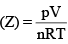versus p, for three real gases A, B and C. Identify the only incorrect statement: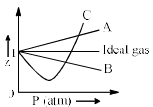Solution:
*Multiple options can be correct
QUESTION: 3

### A gas described by van der Waals’ equation:

Solution:

For a real gas

(P + an2/V2)(V – nb) = nRT

1. Volume large => a=0 and V-nb = V. Hence it reduces to PV = nRT

2. P large but V can’t be neglected. So, P(V-nb) = nRT

3. a and b are temperature independent.

4. For real gas a is not 0 but ideal gas has a = 0. Hence ideal gas exerts more pressure on the container walls.

From the above discussion, A, B and D are correct.

*Multiple options can be correct
QUESTION: 4

According to kinetic theory of gases:

Solution:
*Multiple options can be correct
QUESTION: 5

The root mean square speeds at STP for the gases H2, N2, O2 and HBr are in the order

Solution:
*Multiple options can be correct
QUESTION: 6

Which of the following are same for all ideal gas at same STP?

Solution:
*Multiple options can be correct
QUESTION: 7

Rate of effusion of a gas depends upon:

Solution: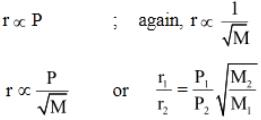*Multiple options can be correct
QUESTION: 8

Which of the following statements are correct?

Solution:
*Multiple options can be correct
QUESTION: 9

Which of the following plots represents ideal gas laws:

Solution:
*Multiple options can be correct
QUESTION: 10

Select the correct statements:

Solution:
*Answer can only contain numeric values
QUESTION: 11

4.215 g of a metallic carbonate was heated in a hard glass tube, the CO2 evolved was found to measure 1336 mL at 27°C and 700 nm of Hg pressure. What is the equivalent weight of the metal:

Solution:
*Answer can only contain numeric values
QUESTION: 12

3.7 g of a gas at 25°C occupied the same volume as 0.184 g of hydrogen at 17°C and at the same pressure. What is the molecular weight of the glass:

Solution:
QUESTION: 13

A hydrocarbon contains 10.5 g of carbon per gram of hydrogen. 1 L of the vapour of the hydrocarbon at 127°C and 1 atm pressure weights 2.8 g. Find the molecular formula of the hydrocarbon.

Solution:
*Answer can only contain numeric values
QUESTION: 14

The pressure in a bulb dropped from 2000 to 1500 mm of mercury in 47 min when the contained oxygen leaked through a small hole. The bulb was then evacuated. A mixture of oxygen and another gas of molecular weight 79 in the molar ratio of 1 : 1 at a total pressure of 4000 mm of mercury was introduced. Find the molar ratio of the two gases remaining in the bulb after a period of 74 min.

Solution:

The change of pressure of oxygen in 47 min is 500 mm Hg. The change of pressure of oxygen after 74 min is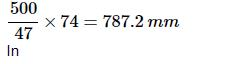the 1:1 molar ratio mixture of oxygen and another gas, each of them has

an equal pressure of 2000 mm because the total pressure is given to be

4000 mm Hg.
The pressure of oxygen left after 74 min is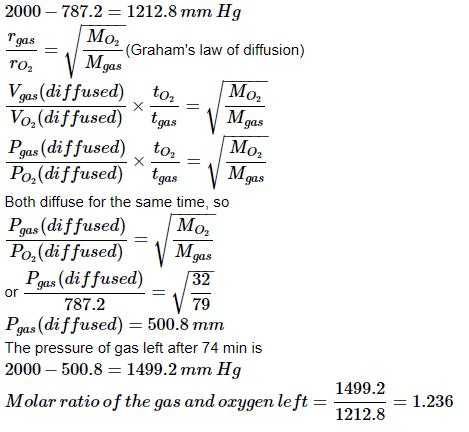QUESTION: 15

Calculate the average kinetic energy, in Joule per molecule in 8.0 g of methane at 27°C:

Solution:

Kinetic energy per molecule of a gas: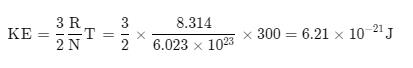*Answer can only contain numeric values
QUESTION: 16

At room temperature, ammonium gas at 1 atm pressure and hydrogen chloride gas at p atm pressure are allowed to effuse through identical pin holes from opposite ends of a glass tube of one metre length and of uniform cross-sect ion. Ammonium chloride is first formed at a distance of 60 cm from the end through which HCl gas is sent in. What is the value of p:

Solution: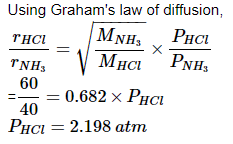*Answer can only contain numeric values
QUESTION: 17

Calculate the pressure exerted by one mole of CO2 gas at 273 K, if the van der Waals’ constant ‘a’ = 3.592 dm6 atm mol–2. Assume that the volume occupied by CO2 molecules is negligible.

Solution:

According to the Van der Waal’s equation we have for one mole of a gas:

[P + a/V2] (V - b) = RT ….(1)

We have

R = 0.082 , T = 273K, V = 22.4 l for 1 mole of an ideal gas at 1 atm pressure.

[P + (3.592 L2 atm/mol2 ) (1.0 mol)2 /(22.4 L)2 ] [22.4 L – (1.0 mol)(0.04267 L/mol)] = (1.0 mol)(0.08206 L atm/mol K)(0+273 K)

P = 0.082 *273/22.4 – 3.592/(22.4)4

P=0.9922 atm

*Answer can only contain numeric values
QUESTION: 18

When 2 g of a gas A is introduced into an evacuated flask kept at 25°C, the pressure is found to be one atmosphere. If 3 g of another gas B is then added to the same flask, the total pressure becomes 1.5 atm. Assuming ideal gas behaviour, calculate the ratio of the molecule weights MA : MB.

Solution:

we know that pressure is proportional to the number of moles present.
when pressure was 1 atm, no. of moles = 2/a moles
when pressure was 1.5 atm, no. of moles = (2/a + 3/b) moles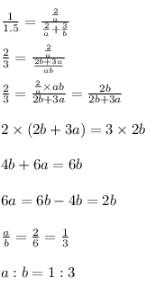QUESTION: 19

Oxygen is present in one litre flask at a pressure of 7.6 × 10–10 mm Hg. Calculate the number of oxygen molecules in the flask at 0°C.

Solution: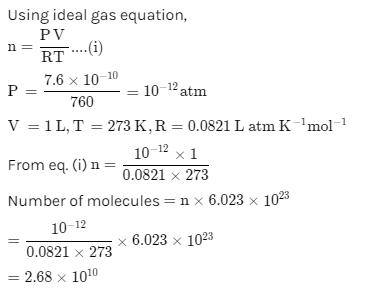*Answer can only contain numeric values
QUESTION: 20

Calculate the root mean square velocity in cm/s of ozone kept in a closed vessel at 20°C and 82 cm mercury pressure.

Solution: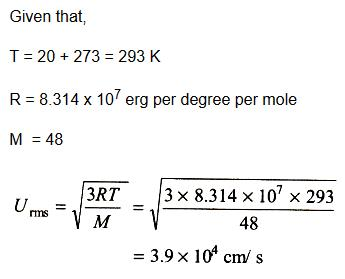*Answer can only contain numeric values
QUESTION: 21

A spherical balloon of 21 cm diameter is to be filled up with hydrogen at NTP from a cylinder containing the gas at 20 atmospheres at 27°C. If the cylinder can hold 2.82 L of water, calculate the number of balloons that can be filled up.

Solution: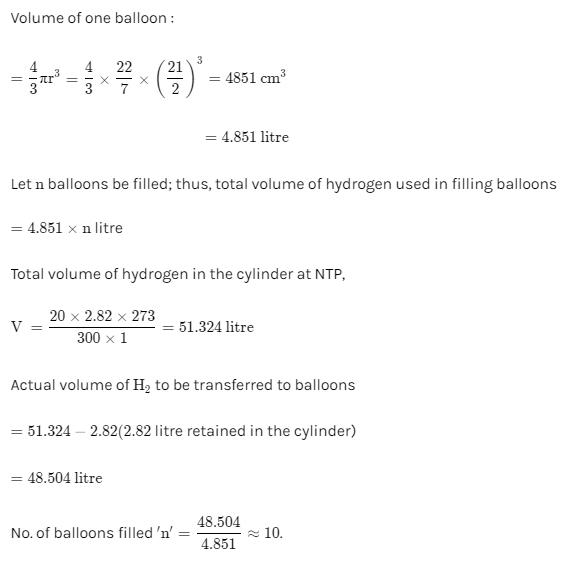*Answer can only contain numeric values
QUESTION: 22

A 4 : 1 molar mixture of He and CH4 is contained in a vessel at 20 bar pressure. Due to a hole in the vessel, the gas mixture leaks out. What is the composition of the mixture effusing out initially.

Solution:

Total moles = 4+1 =5, Total pressure = 20 bars
So, partial pressure of He = 4/5 x 20 = 16 bar
Or, partial pressure of CH4 = 20-16 = 4 bar
Now, molar mass of He = 4, molar mass of CH4 = 16

So, ratio of rate of effusion of He (rHe) and rate of effusion of methane (rCH4) will be: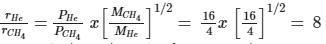Hence, as the rate of effusion of He is 8 times higher than CH4 so, initial mixture must contain the molar ratio of He: CH4​ to be 8:1.

*Answer can only contain numeric values
QUESTION: 23

At 27°C, hydrogen is leaked through a tiny hole into a vessel for 20 min. Another unknown gas at the same temperature and pressure as that of hydrogen is leaked through same bole for 20 min. After the effusion of the gases the mixture exerts a pressure of 6 atm. The hydrogen content of the mixture is 0.7 mole. If the volume of the container is 3 L. What is the molecular weight of the unknown gas?

Solution:
*Answer can only contain numeric values
QUESTION: 24

A mixture of ethene (C2H6) and ethene (C2H4) occupies 40 L at 1.00 atm and at 400 K. The mixture reacts completely with 130 g of O2 to produces CO2 and H2O. Assuming ideal gas behaviour, Calculate the mole fractions of C2H4 and C2H6 in the mixture.

Solution: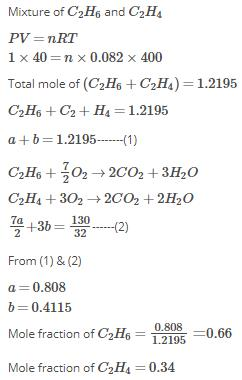QUESTION: 25

In the gas phase, the ratio of excluded volume to molecule volume for a spherical molecule is……………

Solution:
*Answer can only contain numeric values
QUESTION: 26

In an ideal monoatomic gas, the speed of sound is given by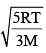. If the speed of sound in argon at 25°C us 1245 km h–1, the root mean square velocity in ms–1 is…………….

Solution:

From kinetic theory of ideal gas,

vs= sqrt[ 5RT/3M]

But,M=mxNo, where m is mass of Argon atom and No is Avogadro number.

Also, R= kNo, where k is Boltzmann constant. Therefore ,

vs=sqrt[ 5 No kT/3mNo]=sqrt[5kT/3m]……(1)

But, (1/2)m<v2 > =(3/2)kT OR

kT=m<v2>/3. Using this in equation (1),

vs= sqrt[5m<v2>/9m]= sqrt[(5/9)<v2] OR

Sqrt(<v2>)=vs x sqrt(9/5)=1245x(5/18)xsqrt(9/5) OR

v rms= 463.9 m/s.

*Answer can only contain numeric values
QUESTION: 27

A stream of oxygen molecules at 500 K exists from a pin-hole in an over and strikes a slit that selects the molecules travelling in a specific direction. Given that the pressure outside the over is 2.5 × 10–7 atm, estimate the maximum distance at which the slit must be placed from the pin-hole in order to produce a collimated beam to oxygen, (Radius of O2 = 1.8 × 10–10 m).

Solution:
*Answer can only contain numeric values
QUESTION: 28

The compression factor (compressibility factor) for one mole of a van der Waal’s at 0oC and 100 atmospheric pressure is found to be 0.5. Assuming that the volume of a gas molecule is negligible, calculate the van der Waals constant a.

Solution:

We know that, Compressibility factor, Z = PV/RT

0.5 = 100 *V/0.082 *273

∴ V = 0.1117L

NOTE : Further when volume of a gas molecule is negligible, van der Waal’s equation becomes

(P + a/V2) (V - 0) = RT

Or PV = RT – a/V or a = RTV – PV2

Substituting the values

A = (0.082 * 0.1119 *273) – (100 * 0.1119 * 0.1119)

= 1.253 atm L2 mol-2

*Answer can only contain numeric values
QUESTION: 29

At 400 K, the root mean square (rms) speed of a gas X (molecule weight = 40) is equal is equal to the most probable speed of gas Y at 60 K. The mo lecular weight of the gas Y is:

Solution:
*Answer can only contain numeric values
QUESTION: 30

Using van der Waals’ equation, calculate the constant ‘a’ when two moles of a gas confined in a four litre flask exert a pressure of 11.0 atm at a temperature of 300 K. The value of ‘b’ is 0.05 L mol–1:

Solution: